# >Cross-Curricular Contexts

## Using spreadsheets within a Mathematical context

I have always wanted to ensure that what I deliver in class is useful and has an authentic context. As part of introducing Digital Literacy skills to the students at school, I have identified an area that is a great 'leveler' in terms of ability. The use of spreadsheets as a cross-curricular tool is a great example of taking learning that can be pretty boring and using it in a fun and relevant way. I also feel this could be a great way to give a boost to those students that lack the confidence in some areas of their Mathematics as well as support those students who thrive in this area.

Patterns, Rules, Fill Series, Absolute and Relative Cell Referencing, Functions...

This lesson is what I always start with when introducing working on spreadsheets. My philosophy around most of what I teach is all about 'Working Smart, Not Hard'. There are some fantastic spreadsheet shortcuts that students can find useful so I feel that this is a great thing to do in order to get them started.

As an aside, this lesson actually has a history. It was the first lesson that I taught as a trainee teacher over 20 years ago. I only occurred to me when I went to search for the demo spreadsheet I had made and saved somewhere in my Google Drive as I have copied a version of it from an old hard drive full of old files from teaching as I know at some point, I won't have to reinvent the wheel and make a resource from scratch.

### Patterns, rules and filling a series

Filling in a spreadsheet is made easier when you know how to use the fill handle.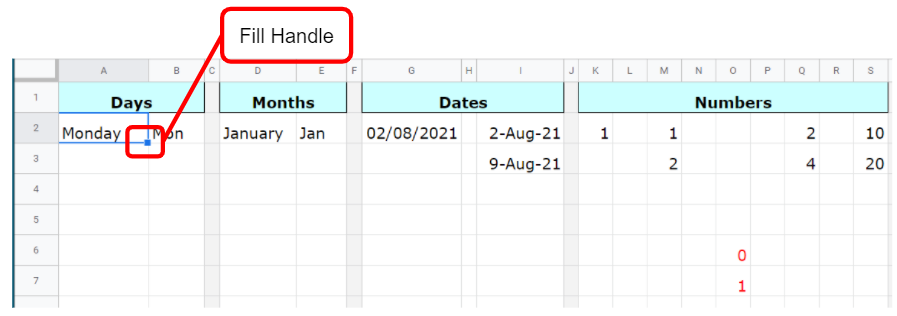Days, months and dates are automatically programmed into the software so these values are automatically filled in when you drag down or across. This works for dates and numbers too. However, to count in weeks or other intervals, the students learn that they need the first two values so they can 'teach' the spreadsheet how to count. This is the same rule for numbers. If you drag down or to the right, the values increase and if you drag up or to the left, the numbers decrease. This means negative values can be created easily.

### Relative & Absolute Cell Referencing

The next stage is demonstrate how the spreadsheet calculates numbers using relative and absolute cell referencing.

Relative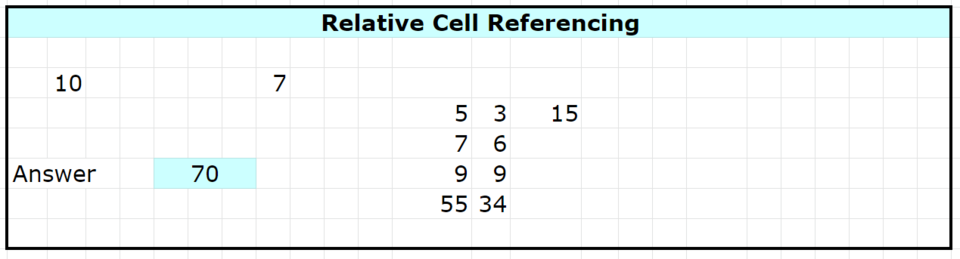When a formula is entered, the two numbers become 'related'.

When a formula is entered, the two numbers become 'related'.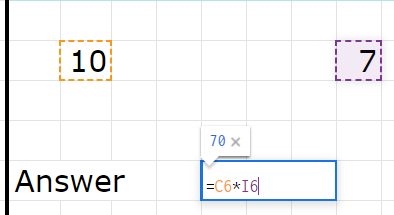If one of these numbers move, the relationship still exists. The example I always use to explain this in class is as follows.

'Imagine the number 7 is me and the number 10 is my family in London. No matter where we are on the world, we are always going to be related.'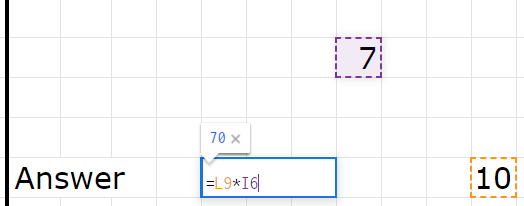I now want to multiply the numbers in the first column below with the second column.The formula is placed in the third column.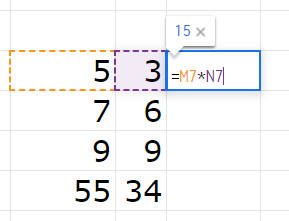The formula can then be copied down to the cells below by using the blue fill handle...

Absolute

Similar to what happens with relative, when a formula is entered, the two numbers become related. However, the difference with absolute is with the dollar signs within the formula.

Note: The dollar sign within a formula has nothing to do with money!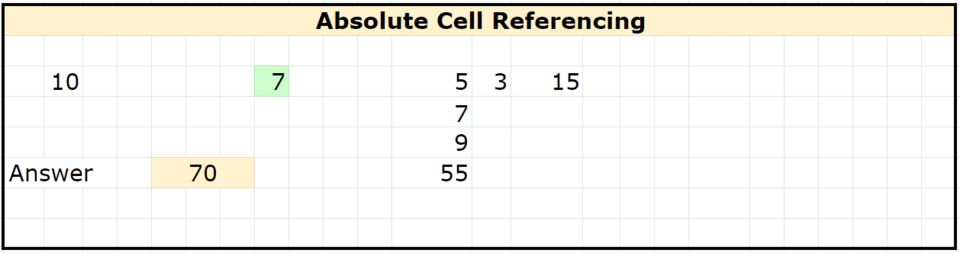In the same way that the relative formula changes when you move the two numbers, the absolute value also changes.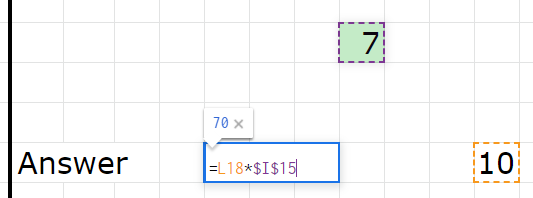If the dollar sign is placed in front of the letter, the value of the cell to be used can be taken anywhere from the column, which in this example is column I.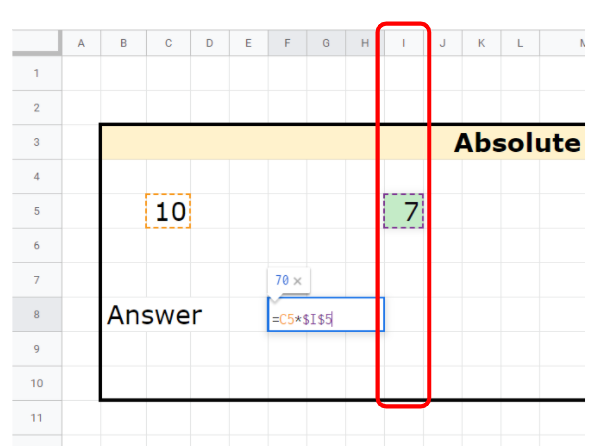If the dollar sign is placed in front of the number, the value of the cell to be used can be taken anywhere from the row, which in this example is row 5.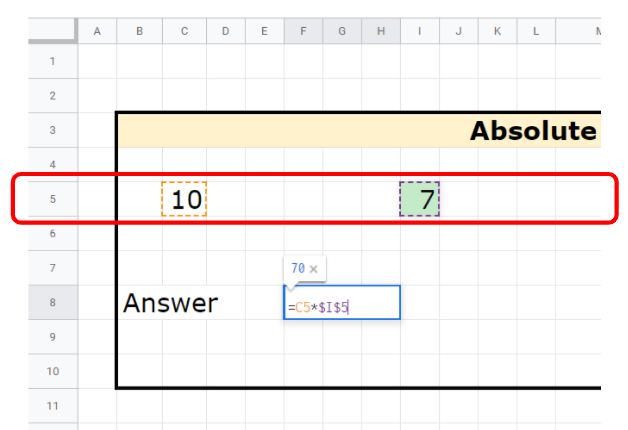If the dollar sign is placed in front of the letter and the number, the focus is on both the column and row. So, in this example the only place that the value can be taken from is cell I5.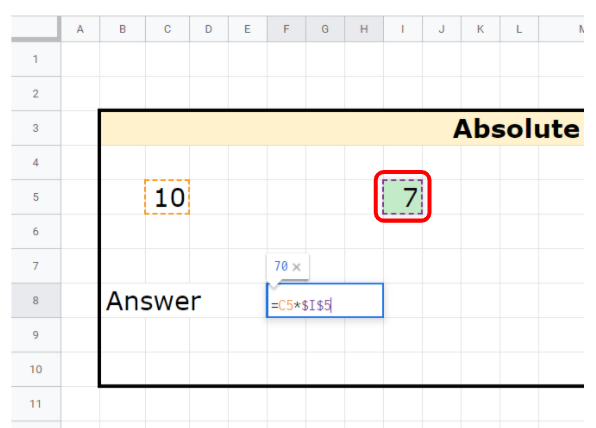I now want to multiply the numbers in the first column with the number 3 in the second column. If I use the fill handle to copy the formula down without using dollar signs, I will get zeros as the answer.

### Times Tables

Now the students have this information, I challenge them to make a Times Table using Relative referencing as quickly as they can.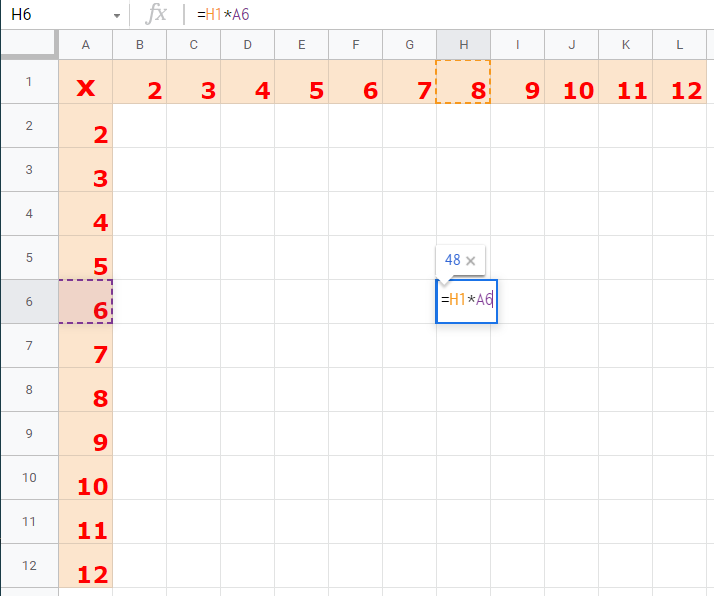Most of the students quickly realise that they cannot use the Fill handle to simply copy the formulas up, down and across as easily as they think...

After a while, I ask the students to see if they can use Absolute referencing and use the Fill handle to make the table in less than two minutes. Some students have already worked this out. It is great to see the look on their faces when they realise how easy it is to complete the challenge!

Now that these features have been explored, the final part of the lesson covers the common functions that can save some time.

• Sum - the sum of a series of numbers or cells
• Average - the average of a series of numbers or cells
• Min
• Max
• Count
• Mode
• CountBlank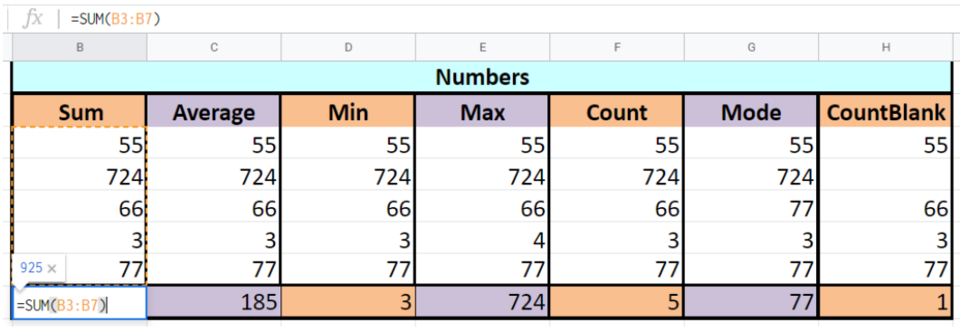The basic rule is to use the values within the selected range to calculate. Some of these functions are used in these follow-up lessons.

## Challenge - Roll The Dice

The challenge is to design and build a spreadsheet to represent two dice being rolled. This is using the functions =RANDBETWEEN to generate a random number from 1 to 6 and =if to display a character according to the value of the random number.

The dice is created with the positions of the dots on the dice as shown.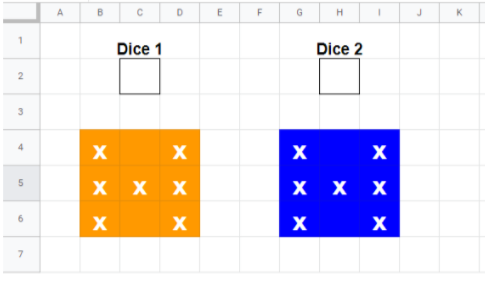The formula =RANDBETWEEN(1,6) goes underneath the labels Dice 1 and Dice 2.

The next part of the challenge is to write the formula for the middle cell.

### =if(OR(\$C\$2=1,\$C\$2=3,\$C\$2=5),"X"," ")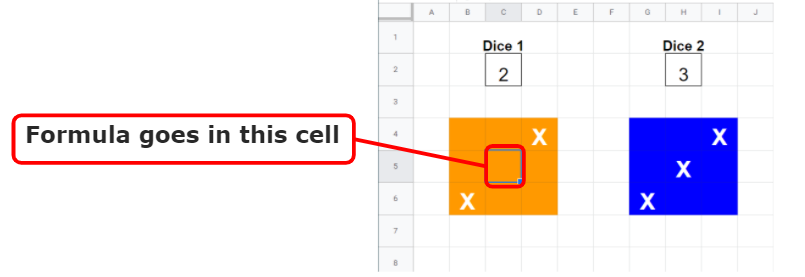The students work through each dot position and place a different version of a formula in each of the cells so that the right number is displayed on each dice.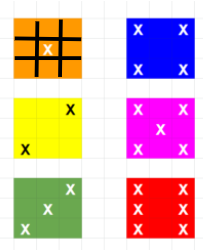Once this is completed, the spreadsheet can be adapted for use, f\or example, as a way to demonstrate Probability in Maths. This spreadsheet was adapted by adding another dice.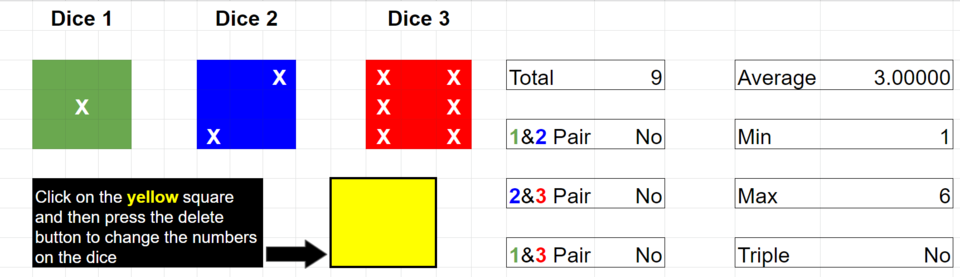The idea is to roll the dice 100 times to find the most common of the following variables:

1. Total
2. Minimum
3. Maximum
4. Pairs
5. Triples
6. Average

This task has the flexibility to be 'low floor, high ceiling', thus enabling students to have a rich mathematical enquiry.

## Challenge - Monster Munch

One of my colleagues wanted to adapt the learning with the class by linking to this Mathematics problem they got from this NZ Maths resource.

https://nzmaths.co.nz/resource/monster-munch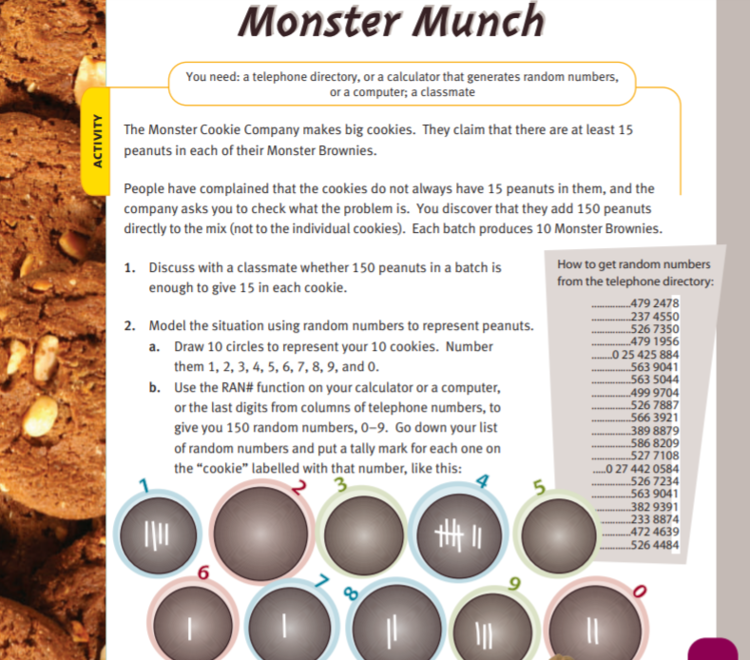This activity explores natural variability and introduces students to the concepts of randomness through mathematical modelling.

The Monster Cookie Company makes big cookies. They claim that there are at least 15 peanuts in each of their Monster Brownies. People have complained that the cookies do not always have 15 peanuts in them, and the company asks you to check what the problem is. You discover that they add 150 peanuts directly to the mix (not to the individual cookies). Each batch produces 10 Monster Brownies.

So the students were asked to create a spreadsheet that would generate random numbers to model what might happen in the making of the cookies. To start, the students were asked to make a template looking a little bit like this: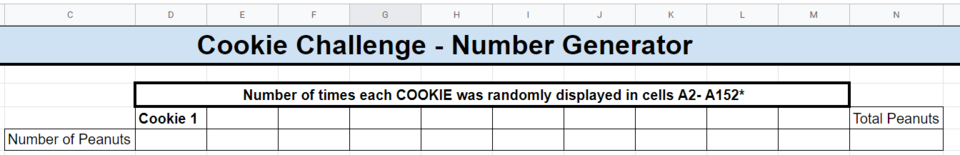The next stage is to generate a random number to represent each cookie. This is using the function =RANDBETWEEN to generate a random number from 1 to 10.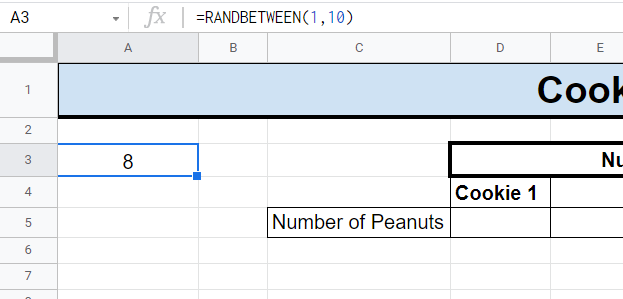Use the fill handle to copy this formula down the column until there are a total of 150 random numbers to represent the amount of peanuts in the cookie mix.

The next step is to count how many peanuts are randomly generated for each cookie. To do this, the function =COUNTIF can be used to count how many of each of the numbers 1 to 10 appear in the list of 150 randomly generated numbers. In this case, the formula =COUNTIF(A3:A152,1) is used for Cookie 1. The formula counts how many 1s appear in the list.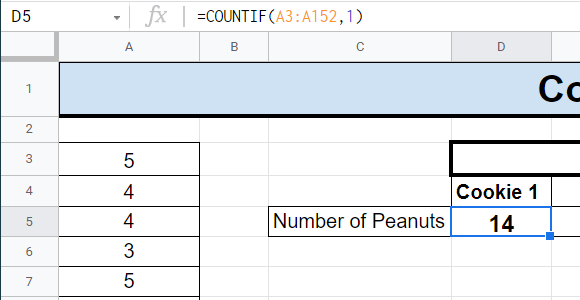The formula =COUNTIF(A3:A152,2) is used for Cookie 2 to count how many 2s appear in the list. You will see the last number in the formula changes as it corresponds with the cookie number.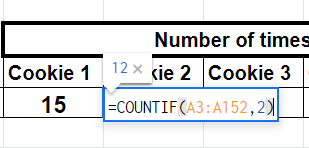This process is repeated until all ten cookies have a corresponding formula.